Students can Download Maths Chapter 1 Number System Intext Questions and Answers, Notes Pdf, Samacheer Kalvi 7th Maths Book Solutions Guide Pdf helps you to revise the complete Tamilnadu State Board New Syllabus and score more marks in your examinations.

## Tamilnadu Samacheer Kalvi 7th Maths Solutions Term 2 Chapter 1 Number System Intext Questions

Exercise 1.1
(Try These Text book Page No. 2)

7th Maths Term 2 Exercise 1.1 Question 1.
Observe the following and write the fraction of the shaded portion and mention in decimal form also.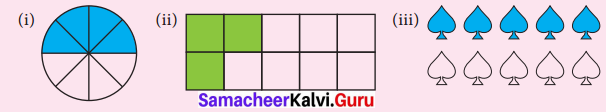Solution:
(i) Total parts = 8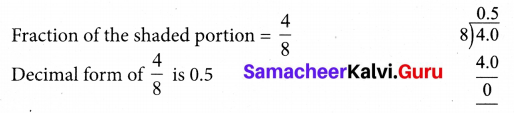(ii)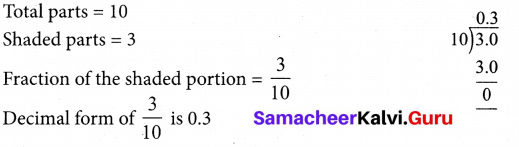(iii)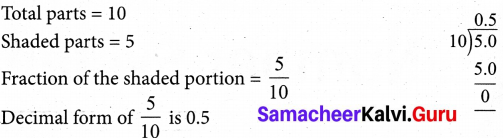Samacheer Kalvi 7th Maths Book Answers Term 2 Question 2.
Represent the following fractions in decimal form by converting denominator into ten or powers of 10.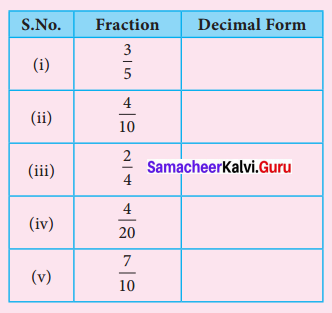Solution: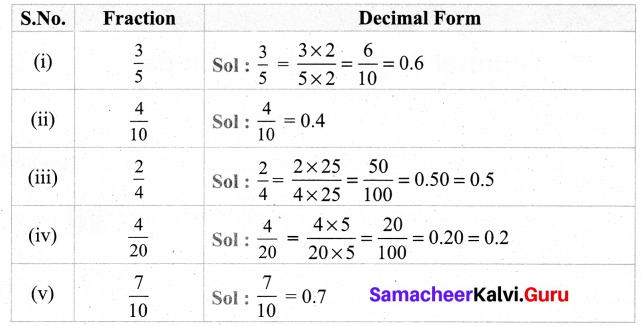Samacheer Kalvi 7th Maths Book Solutions Term 2 Question 3.
Give any two life situations where we use decimal numbers.
Solution:
(i) Measuring weight of gold.
(ii) Weighing our height

(Try These Text book Page No. 3)

7th Std Samacheer Kalvi Maths Solutions Term 2 Question 1.
Represent the following decimal numbers pictorially.
(i) 5 ones and 3 tenths
(ii) 6 tenths
(iii) 7 ones and 9 tenths
(iv) 6 ones and 4 tenths
(v) Seven tenths
Solution:
(i) 5 ones and 3 tenths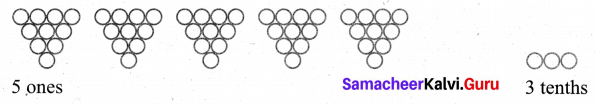(ii) 6 tenths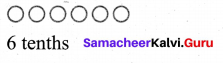(iii) 7 ones and 9 tenths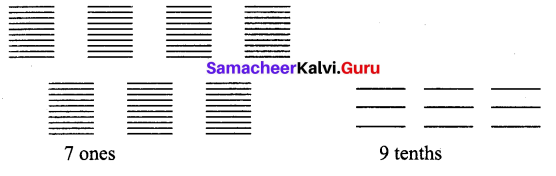(iv) 6 ones and 4 tenths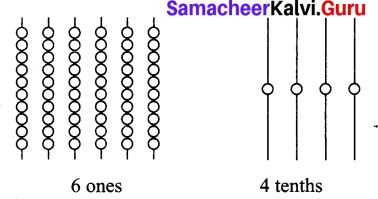(v) Seven tenths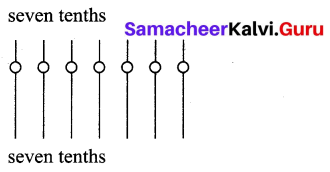(Try These Text book Page No. 5 & 6)

7th Standard Maths Number System Exercise 1.1 Question 1.
Express the following decimal numbers in an expanded form and place value grid form.
(i) 56.78
(ii) 123.32
(iii) 354.56
Solution:
(i) 56.78
(a) Expanded form
56.78 = 5 × 101 + 6 × 100 + 7 × 10-1 + 8 × 10-2

(b) Place value grid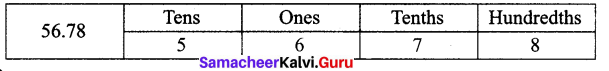(ii) 123.32
(a) Expanded form
123.32 = 1 × 102 + 2 × 101 + 3 × 100 + 3 × 10-1 +2 × 10-2

(b) Place value grid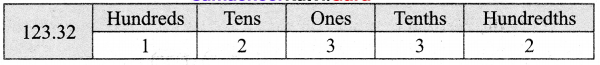(iii) 354.56
(a) Expande form
354.56 = 3 × 102 + 5 × 101 + 4 × 100+ 5 × 10-1 + 6 × 10-2

(b) Place value grid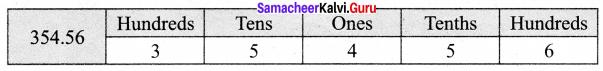7th Maths Exercise 1.1 Samacheer Kalvi Question 2.
Express the following measurements in terms of metre and in decimal form. One is done for you.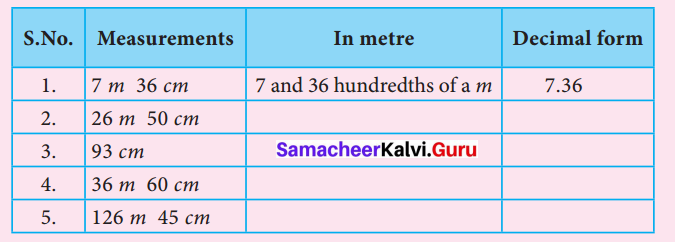Solution: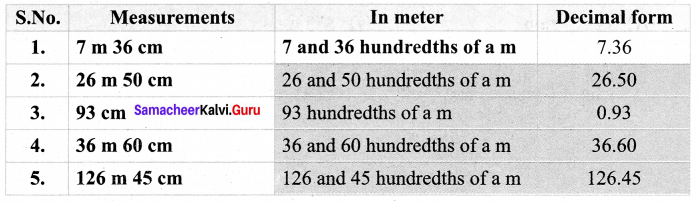Samacheer Kalvi 7th Maths Term 2 Question 3.
Write the following numbers in the place value grid and find the place value of the underlined digits.
(i) 36.37
(ii) 267.06
(iii) 0.23
(iv) 27.69
(v) 53.27
Solution: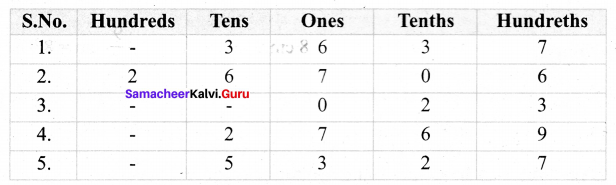(i) Place value of 3 in 36.37 is Tenths.
(ii) Place value of 6 in 267.06 is Hundredths.
(iii) Place value of 2 in 0.23 is Tenths.
(iv) Place value of 9 in 27.69 is Hundredths.
(v) Place value of 2 in 53.27 is Tenths.

Exercise 1.2

(Try These Text book Page No. 10)

7th Maths Guide Try These Question 1.
Convert the following fractions into the decimal numbers.
(i) $$\frac { 16 }{ 1000 }$$
(ii) $$\frac { 638 }{ 10 }$$
(iii) $$\frac { 1 }{ 20 }$$
(iv) $$\frac { 3 }{ 50 }$$
Solution:
(i) $$\frac { 16 }{ 1000 }$$ = 0.016
(ii) $$\frac { 638 }{ 10 }$$ = 63.8
(iii) $$\frac { 1 }{ 20 }$$ = $$\frac{1 \times 5}{20 \times 5}$$ = $$\frac { 5 }{ 100 }$$ = 0.05
(iv) $$\frac { 3 }{ 50 }$$ = $$\frac{3 \times 2}{50 \times 2}$$ = $$\frac { 6 }{ 100 }$$ = 0.06

Samacheer Kalvi 7th Maths Book Answers Question 2.
Write the fraction for each of the following:
(i) 6 hundreds + 3 tens + 3 ones + 6 hundredths + 3 thousandths.
(ii) 3 thousands + 3 hundreds + 4 tens + 9 ones + 6 tenths.
Solution:
(i) 6 hundreds + 3 tens + 3 ones + 6 hundreds + 3 thousandths.
= 6 × 100 + 3 × 10 + 3 × 1 + 0 × $$\frac { 1 }{ 10 }$$ + 6 × $$\frac { 1 }{ 100 }$$ + 3 × $$\frac { 1 }{ 1000 }$$
= 600 + 30 + 3 + 0 + $$\frac { 6 }{ 100 }$$ + $$\frac { 3 }{ 1000 }$$
= 633 + 0.06 + 0.003
= 633.063

(ii) 3 thousands + 3 hundreds + 4 tens + 9 ones + 6 tenths.
= 3 × 1000 + 3 × 100 + 4 × 10 + 9 × 1 + 6 × $$\frac { 1 }{ 10 }$$
= 3000 + 300 + 40 + 9 + $$\frac { 6 }{ 10 }$$
= 3349 + 0.6
= 3349.6

7th Std Maths Guide Question 3.
Convert the following decimals into fractions.
(i) 0.0005
(ii) 6.24
Solution:
(i) 0.0005 = $$\frac { 5 }{ 10000 }$$ = $$\frac{5 \div 5}{10000 \div 5}$$ = $$\frac { 1 }{ 2000 }$$
(ii) 6.24 = $$\frac { 624 }{ 100 }$$ = $$\frac{624 \div 4}{100 \div 4}$$ = $$\frac { 156 }{ 25 }$$

Exercise 1.4

(Try These Text book Page No. 22)

Samacheer Kalvi 7th Term 2 Maths Solutions Question 1.
Mark the following decimal numbers on the number line.
(i) 0.3
(ii) 1.7
(iii) 2.3
Solution:
(i) 0.3
We know that 0.3 is more than 0, but less than 1.
There are 3 tenths in it. Divide the unit lenght between O and 1 on the number line
into 10 equal parts and take 3 parts, which represent 0.3.(ii) 1.7
We know that 1.7 is more than 1, but less than 2.
There are one ones and 7 tenths in it. Divide the unit length between 1 and 2 on the number line into 10 equal parts and take 7 parts which represents 1.7 = 1 + 0.7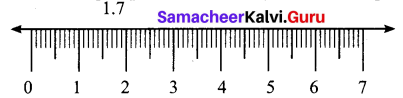(iii) We know that 2.3 is more than 2 and less than 3.
There are 2 ones and 3 tenths in it. Divide the unit length between 2 and 3 into 10 equal parts and take 3 parts, which represents 2.3 = 2 + 0.3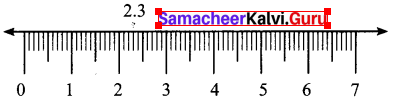Samacheer Kalvi 7 Maths Question 2.
Identify any two decimal numbers between 2 and 3.
Solution:
2.5 and 2.9

Samacheer Kalvi 7th Standard Maths Question 3.
Write any decimal number which is greater than 1 and less than 2.
Solution:
1.7, 1.9, 1.6, ………………..